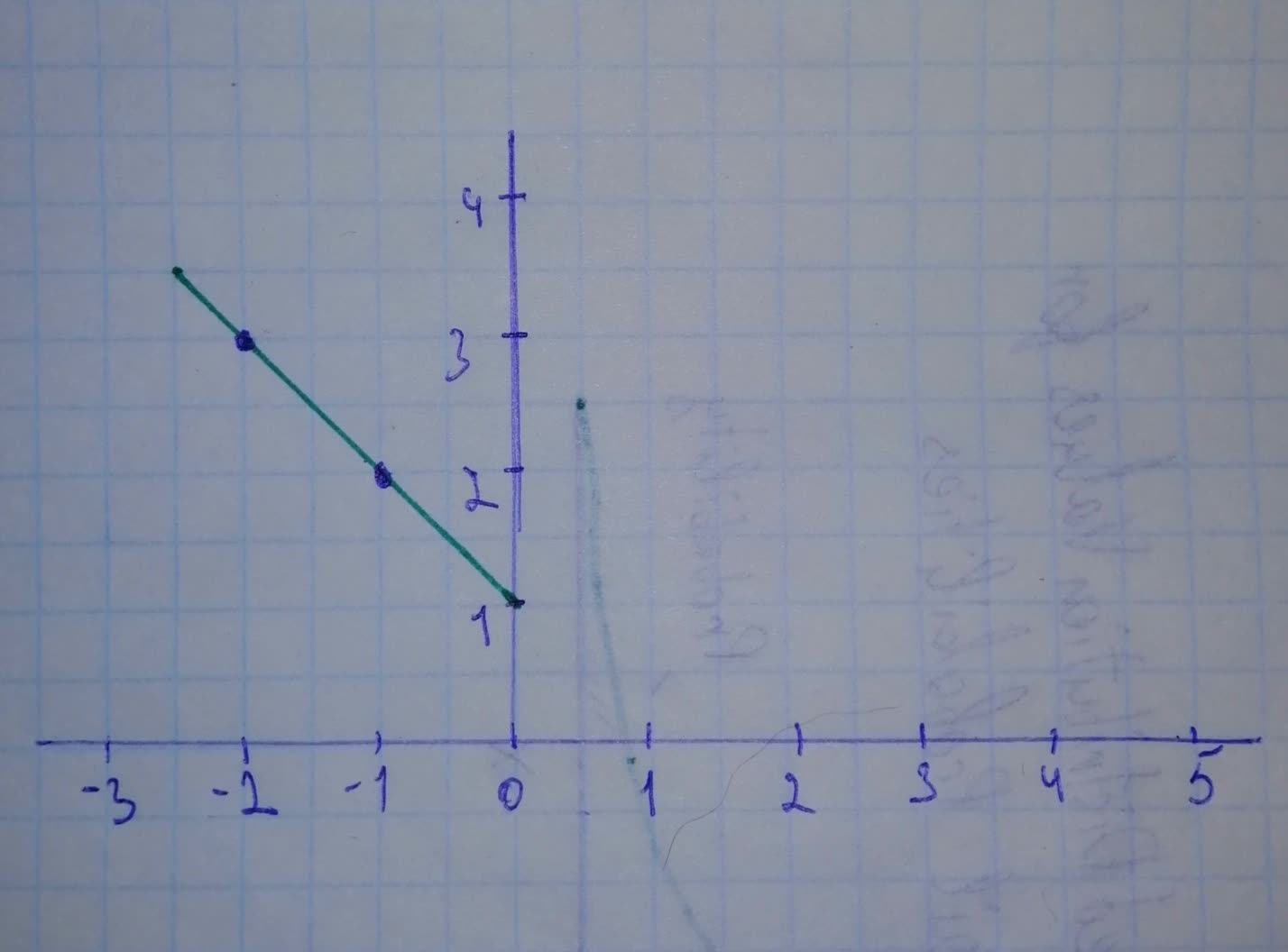Sketch a graph of the function. Use transformations of functions whenever possibEmily-Jane Bray 2021-09-19 Answered

Sketch a graph of the function. Use transformations of functions whenever possible.
$$f(x)=\begin{cases}1-x & if & x<0 \\1 & if & x\geq0\end{cases}$$

• Questions are typically answered in as fast as 30 minutes

Solve your problem for the price of one coffee

• Math expert for every subject
• Pay only if we can solve itbahaistag

Step 1
Function is defined partially on intervals.
When $$\displaystyle{x}{<}{0}$$, funktion is linear, so create table of values and graph a line through two points:
$$\begin{array}{|c|c|}\hline x & f(x) \\ \hline -2 & 3 \\ \hline -1 & 2 \\ \hline \end{array}$$
When $$\displaystyle{x}\geq{0}$$ , function is constant, so graph horizontal line $$\displaystyle{y}={1}$$.